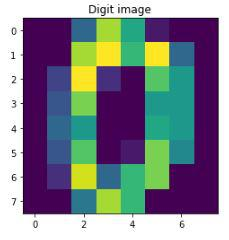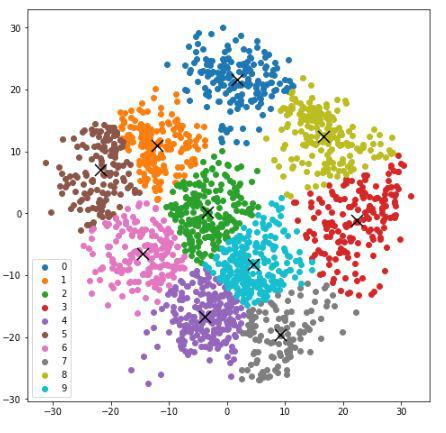GeeksforGeeks App
Open AppBrowser
Continue

# K-Means clustering on the handwritten digits data using Scikit Learn in Python

K – means clustering is an unsupervised algorithm that is used in customer segmentation applications. In this algorithm, we try to form clusters within our datasets that are closely related to each other in a high-dimensional space.

In this article, we will see how to use the k means algorithm to identify the clusters of the digits.

## Python3

 `from` `sklearn.datasets ``import` `load_digits`` ` `digits_data ``=` `load_digits().data`

Output:

```array([[ 0.,  0.,  5., ...,  0.,  0.,  0.],
[ 0.,  0.,  0., ..., 10.,  0.,  0.],
[ 0.,  0.,  0., ..., 16.,  9.,  0.],
...,
[ 0.,  0.,  1., ...,  6.,  0.,  0.],
[ 0.,  0.,  2., ..., 12.,  0.,  0.],
[ 0.,  0., 10., ..., 12.,  1.,  0.]])```

Each handwritten digit in the data is an array of color values of pixels of its image. For better understanding, let’s print how the data of the first digit looks like and then display its’s respective image

## Python3

 `import` `matplotlib.pyplot as plt`` ` `print``(``"First handwritten digit data: "` `+` `digits_data[``0``])``sample_digit ``=` `digits_data[``0``].reshape(``8``, ``8``)``plt.imshow(sample_digit)``plt.title(``"Digit image"``)``plt.show()`

Output:

First handwritten digit data:  [ 0.  0.  5. 13.  9.  1.  0.  0.  0.  0. 13. 15. 10. 15.  5.  0.  0.  3.

15.  2.  0. 11.  8.  0.  0.  4. 12.  0.  0.  8.  8.  0.  0.  5.  8.  0.

0.  9.  8.  0.  0.  4. 11.  0.  1. 12.  7.  0.  0.  2. 14.  5. 10. 12.

0.  0.  0.  0.  6. 13. 10.  0.  0.  0.]Sample image from the dataset

In the next step, we scale the data. Scaling is an optional yet very helpful technique for the faster processing of the model. In our model, we scale the pixel values which are typically between 0 – 255 to -1 – 1, easing the computation and avoiding super large numbers. Another point to consider is that a train test split is not required for this model as it is unsupervised learning with no labels to test. Then, we define the k value, which is 10 as we have 0-9 digits in our data. Also setting up the target variable.

## Python3

 `from` `sklearn.preprocessing ``import` `scale`` ` `scaled_data ``=` `scale(digits_data)``print``(scaled_data)`` ` `Y ``=` `load_digits().target``print``(Y)`

Output:

[[ 0.         -0.33501649 -0.04308102 … -1.14664746 -0.5056698

-0.19600752]

[ 0.         -0.33501649 -1.09493684 …  0.54856067 -0.5056698

-0.19600752]

[ 0.         -0.33501649 -1.09493684 …  1.56568555  1.6951369

-0.19600752]

…

[ 0.         -0.33501649 -0.88456568 … -0.12952258 -0.5056698

-0.19600752]

[ 0.         -0.33501649 -0.67419451 …  0.8876023  -0.5056698

-0.19600752]

[ 0.         -0.33501649  1.00877481 …  0.8876023  -0.26113572

-0.19600752]]

[0 1 2 … 8 9 8]

## Defining k-means clustering:

Now we define the K-means cluster using the KMeans function from the sklearn module.

### Method 1: Using a Random initial cluster.

• Setting the initial cluster points as random data points by using the ‘init‘ argument.
• The argument ‘n_init‘ is the number of iterations the k-means clustering should run with different initial clusters chosen at random, in the end, the clustering with the least total variance is considered’
• The random state is kept to 0 (any number can be given) to fix the same random initial clusters every time the code is run.

## Python3

 `from` `sklearn.cluster ``import` `KMeans`` ` `k ``=` `10``kmeans_cluster ``=` `KMeans(init ``=` `"random"``,``                        ``n_clusters ``=` `k,``                        ``n_init ``=` `10``,``                        ``random_state ``=` `0``)`

### Method 2: Using k-means++

It is similar to method-1 however, it is not completely random, and chooses the initial clusters far away from each other. Therefore, it should require fewer iterations in finding the clusters when compared to the random initialization.

## Python3

 `kmeans_cluster ``=` `KMeans(init``=``"k-means++"``, n_clusters``=``k, n_init``=``10``, random_state``=``0``)`

### Model Evaluation

We will use scores like silhouette score,  time taken to reach optimum position, v_measure  and some other important metrics.

## Python3

 `def` `bench_k_means(estimator, name, data):``    ``initial_time ``=` `time()``    ``estimator.fit(data)``    ``print``(``"Initial-cluster: "` `+` `name)``    ``print``(``"Time taken: {0:0.3f}"``.``format``(time() ``-` `initial_time))``    ``print``(``"Homogeneity: {0:0.3f}"``.``format``(``        ``metrics.homogeneity_score(Y, estimator.labels_)))``    ``print``(``"Completeness: {0:0.3f}"``.``format``(``        ``metrics.completeness_score(Y, estimator.labels_)))``    ``print``(``"V_measure: {0:0.3f}"``.``format``(``        ``metrics.v_measure_score(Y, estimator.labels_)))``    ``print``(``"Adjusted random: {0:0.3f}"``.``format``(``        ``metrics.adjusted_rand_score(Y, estimator.labels_)))``    ``print``(``"Adjusted mutual info: {0:0.3f}"``.``format``(``        ``metrics.adjusted_mutual_info_score(Y, estimator.labels_)))``    ``print``(``"Silhouette: {0:0.3f}"``.``format``(metrics.silhouette_score(``        ``data, estimator.labels_, metric``=``'euclidean'``, sample_size``=``300``)))`

We will now use the above helper function to evaluate the performance of our k means algorithm.

## Python3

 `kmeans_cluster ``=` `KMeans(init``=``"random"``, n_clusters``=``k, n_init``=``10``, random_state``=``0``)``bench_k_means(estimator``=``kmeans_cluster, name``=``"random"``, data``=``digits_data)`` ` `kmeans_cluster ``=` `KMeans(init``=``"k-means++"``, n_clusters``=``k,``                        ``n_init``=``10``, random_state``=``0``)``bench_k_means(estimator``=``kmeans_cluster, name``=``"random"``, data``=``digits_data)`

#### Output:

```Initial-cluster: random
Time taken: 0.302
Homogeneity: 0.739
Completeness: 0.748
V_measure: 0.744
Silhouette: 0.191

Initial-cluster: random
Time taken: 0.386
Homogeneity: 0.742
Completeness: 0.751
V_measure: 0.747
Silhouette: 0.175```

### Visualizing the K-means clustering for handwritten data:

• Plotting the k-means cluster using the scatter function provided by the matplotlib module.
• Reducing the large dataset by using Principal Component Analysis (PCA) and fitting it to the previously defined k-means++ model.
• Plotting the clusters with different colors, a centroid was marked for each cluster.

## Python3

 `from` `sklearn.decomposition ``import` `PCA``import` `numpy as np`` ` `# Reducing the dataset``pca ``=` `PCA(``2``)``reduced_data ``=` `pca.fit_transform(digits_data)``kmeans_cluster.fit(reduced_data)`` ` `# Calculating the centroids``centroids ``=` `kmeans_cluster.cluster_centers_``label ``=` `kmeans_cluster.fit_predict(reduced_data)``unique_labels ``=` `np.unique(label)`` ` `# plotting the clusters:``plt.figure(figsize``=``(``8``, ``8``))``for` `i ``in` `unique_labels:``    ``plt.scatter(reduced_data[label ``=``=` `i, ``0``],``                ``reduced_data[label ``=``=` `i, ``1``],``                ``label``=``i)``plt.scatter(centroids[:, ``0``], centroids[:, ``1``],``            ``marker``=``'x'``, s``=``169``, linewidths``=``3``,``            ``color``=``'k'``, zorder``=``10``)``plt.legend()``plt.show()`

#### Output:Clusters of the data points

### Conclusion

From the above graph, we can observe the clusters of the different digits are approximately separable from one another.

My Personal Notes arrow_drop_up